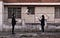Photo by Andrés Gómez on Unsplash

# Password Juggling in Discrete Logs and Elliptic Curves

I love implementing things in discrete logs and then converting them into elliptic curve methods. Basically a exponentiation (g^x mod p) becomes a multiplication (xG), a multiplication (g^x g^y mod p) becomes a point addition (xG+yG), and a division (g^x /g^y mod p) becomes a point subtraction (xG-yG). In this article I will show all three of these operations, and show how we can convert from discrete logs into elliptic curve methods.

J-PAKE (Password Authenticated Key Exchange by Juggling) was created by Hao and Ryan . It is a Password Authentication Key Exchange method, and where Bob and Alice share the same secret password. They can then generate a shared secret key. It involves two stages: a one-time key establishment; and a key confirmation stage. Overall, it does not need access to the PKI infrastructure.

In Round 1, Alice then generates two random values: x_1 and x_2. These are kept secret, and where Alice will send the following to Bob:

Bob then generates two random values: x_3 and x_4. These are kept secret, and where Bob will send the following to Alice:

In Round 2, Alice then calculates the following and sends to Bob:

Bob then calculates the following and sends to Alice:

Alice then calculates the shared key as:

Bob then calculates the shared key as:

The following is the code [here]:

`import sysimport randomimport hashlibfrom Crypto.Util.number import getPrimefrom Crypto.Random import get_random_bytesfrom libnum import invmodprimebits=64secret="Hello"s=int(hashlib.md5(secret.encode()).hexdigest(),16)if (len(sys.argv)>1):  primebits=int(sys.argv)p = getPrime(primebits, randfunc=get_random_bytes)g=3x1 = random.randint(0, p-1)x2 = random.randint(0, p-1)x3 = random.randint(0, p-1)x4 = random.randint(0, p-1)g_x1 = pow(g,x1,p)g_x2 = pow(g,x2,p)g_x3 = pow(g,x3,p)g_x4 = pow(g,x4,p)A=pow(g_x1*g_x3*g_x4,x2*s,p)B=pow(g_x1*g_x2*g_x3,x4*s,p)K_inv1 = invmod(pow(g_x4,x2*s,p),p)K_inv2 = invmod(pow(g_x2,x4*s,p),p)print (f"Password: {secret}")print ("===Alice parameters=== ")print (f"Alice x1={x1}, x2={x2}\nAlice sends: g^x1 (mod p)={g_x1}, g^x2 (mod p)={g_x2}")print ("\n===Bob parameters=== ")print (f"Bob x3={x3}, x4={x4}\nBob sends: g^x3 (mod p)={g_x3}, g^x4 (mod p)={g_x4}")print ("\n===Alice parameters=== ")print (f"Alice sends A={A}")print ("\n===Bob parameters=== ")print (f"Bob sends B={B}")print ("\nKey Alice:\t",pow(B*K_inv1,x2,p))print ("Key Bob:\t",pow(A*K_inv2,x4,p))`

And a sample run [here]::

`Password: Hello===Alice parameters=== Alice x1=157213077345404166232851178443413218352, x2=60882969011129026583507048344883123589Alice sends: g^x1 (mod p)=56781426656681641144186196017500880545, g^x2 (mod p)=121860746084617583915988849727105883560===Bob parameters=== Bob x3=45903653766348112533871178311001724528, x4=1638010860195229639042475084719695992Bob sends: g^x3 (mod p)=132285796655680352374416311434659192709, g^x4 (mod p)=11399416518610155287102460214899329936===Alice parameters=== Alice sends A=106163473460122235652996423416153745041===Bob parameters=== Bob sends B=104256257064667401922110896172192982198Key Alice:	 161131477957450225970071118935386964443Key Bob:	 161131477957450225970071118935386964443`

In Round 1, Alice then generates two random values: x_1 and x_2. These are kept secret, and where Alice will send the following points to Bob:

Bob then generates two random values: x3 and x4. These are kept secret, and where Bob will send the following points to Alice:

In Round 2, Alice then calculates the following point and sends to Bob:

Bob then calculates the following point and sends to Alice:

Alice then calculates the shared key as:

Bob then calculates the shared key as:

The following is the code [here]:

`from secp256k1 import curve,scalar_mult, point_add,point_negimport randomimport hashlibimport syssecret="Hello"if (len(sys.argv)>1):  secret=sys.argvs=int(hashlib.md5(secret.encode()).hexdigest(),16)# Alice generatesx1 = random.randint(0, curve.n-1)x2 = random.randint(0, curve.n-1)# Bob generatesx3 = random.randint(0, curve.n-1)x4 = random.randint(0, curve.n-1)# Alice generatesx1G = scalar_mult(x1, curve.g)x2G = scalar_mult(x2, curve.g)# Alice generatesx3G = scalar_mult(x3, curve.g)x4G = scalar_mult(x4, curve.g)# A= (G1 + G3 + G4) x [x2*s] A = point_add(x1G,x3G)A = point_add(A,x4G)A = scalar_mult(x2*s,A)# B=(G1 + G2 + G3) x [x4*s]B = point_add(x1G,x2G)B = point_add(B,x3G)B = scalar_mult(x4*s,B)# Ka = (B - (G4 x [x2*s])) x [x2]Ka = scalar_mult(x2,point_add(B,point_neg(scalar_mult(x2*s,x4G))))# Bob computes Kb = (A - (G2 x [x4*s])) x [x4]Kb = scalar_mult(x4,point_add(A,point_neg(scalar_mult(x4*s,x2G))))print (f"Shared password: {secret}")print ("===Alice parameters=== ")print (f"Alice x1={x1}, x2={x2}\nAlice sends: x1G ={x1G}, x2G (mod p)={x2G}")print ("\n===Bob parameters=== ")print (f"Bob x3={x3}, x4={x4}\nBob sends: x3G ={x3G}, x4G ={x4G}")print ("\n===Alice parameters=== ")print (f"Alice sends A={A}")print ("\n===Bob parameters=== ")print (f"Bob sends B={B}")print (f"\nAlice key: {Ka}")print (f"Bob key: {Kb}")`

And a sample run [here]:

`Shared password: Hello===Alice parameters=== Alice x1=109223778996008288522752313848072201758184207182558942631021579873936392111849, x2=70164035479348133703302704351481332338501967890693555425529073473167539752301Alice sends: x1G =(25757379941637732465573935070442020365879599102324511094843713901095524531800, 54326101113419731239507117799777645852329796924147840511329613587119920683693), x2G (mod p)=(5706311334803882186027182100991396833153739570993758136649404286549554001570, 106168453431397971102840065735250567879463130661919689523977025175625629858690)===Bob parameters=== Bob x3=113328284219482995795681121841598407747113363163727872101184389718131489417226, x4=94863383611815555242143536561323826445308191135350794049205403770770601196846Bob sends: x3G =(40880339132884486501685633826331351175176673629328173409146139796674827176388, 27794339853952991181191384213539267701325905882975701216294183824555854013681), x4G =(108245762028214747089045785337280106838812776556601588479474228996536995423797, 105254641585474920614907618522803316359119132070853766923246033513721029568275)===Alice parameters=== Alice sends A=(113708982016570409852826497024398419040874810528428747983711214340060038552407, 12449611840362587536436172331378674267418812633125332589127742521395727671735)===Bob parameters=== Bob sends B=(32362610944530295763556764702321503631851749992465430289848515183640872056976, 2507094715922200386410039051112915477205177066245229095569452807229671828742)Alice key: 39828201834688558297506388516640559720610311763003967947405469276609917171305Bob key: 39828201834688558297506388516640559720610311763003967947405469276609917171305`

And that’s it! You can try here:

and:

 Hao, F., & Ryan, P. Y. (2008, April). Password authenticated key exchange by juggling. In International Workshop on Security Protocols (pp. 159–171). Springer, Berlin, Heidelberg [here].

Professor of Cryptography. Serial innovator. Believer in fairness, justice & freedom. EU Citizen. Auld Reekie native. Old World Breaker. New World Creator.

## More from Prof Bill Buchanan OBE

Professor of Cryptography. Serial innovator. Believer in fairness, justice & freedom. EU Citizen. Auld Reekie native. Old World Breaker. New World Creator.